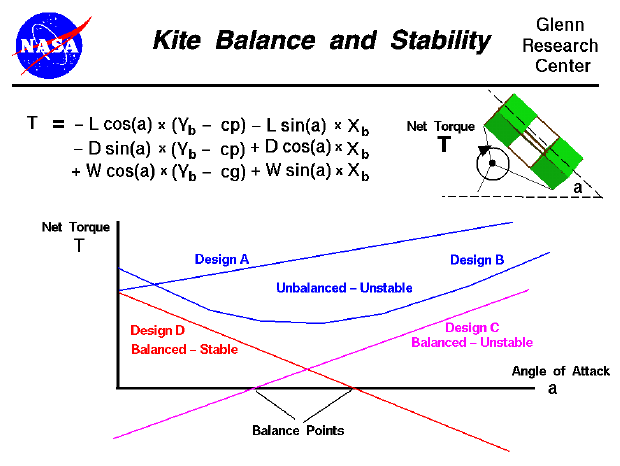Newton's first law of motion states that every object will remain at rest or in uniform motion in a straight line unless compelled to change its state by the action of an external force. In general, an external force produces both a translation and a rotation of an object. The translation is described by Newton's second law of motion applied to the center of gravity, cg, of the object. In general, the external force is applied at some point other than the cg. If the object is unconstrained, like an aircraft in flight, the object rotates about the cg due to the applied torque about the cg. (If the external force is applied at the cg, there is no rotation of an unconstrained object.) If the object is constrained at some point, called the pivot, the object rotates about the pivot due to the torque about the pivot. In equilibrium, or balance, there are no net torques about the pivot and the object does not rotate.

In flight, a kite rotates about the bridle point which is the knot that attaches the control line to the bridle string. The kite rotates because of the torques generated by the weight and the aerodynamic forces. On this page, we show the equation which describes the torque about the bridle point.

T = - L * cos(a) * (yb - cp) - L * sin(a) * xb
- D * sin(a) * (yb - cp) + D * cos(a) * xb
+ W * cos(a) * (yb - cg) + W * sin(a) * xb

where T is the net torque with a positive torque being in the clockwise direction. The forces are: L, the lift, D, the drag, and W, the weight. a is the angle of attack which affects the magnitude of the aerodynamic forces. Xb and Yb are the co-ordinates of the bridle point, cg is the location of the center of gravity and cp is the location of the center of pressure. The values for the weight and the geometric variables are a constant for a particular design. Therefore, the net torque about the bridle point is a function of the angle of attack.

On the slide, we have plotted the net torque versus angle of attack for several different designs. If we can determine an angle of attack which makes the net torque equal to zero, we have a balanced condition and the kite will not rotate in flight. If the plot for a given design does not cross the angle of attack axis, the kite never achieves a balanced condition and will continuously rotate about the bridle point. Unbalanced designs are shown in blue for design A and B. Balanced designs are shown for design C and D with the balance point indicated on the figure. But balancing the kite is only part of the design problem. In flight, the kite experiences small changes in the angle of attack due to turbulence in the air. If the torque increases with a slight increase in angle of attack, we have an unstable condition. The increased torque causes the angle of attack to increase, which causes more torque, which causes more angle of attack .. and the kite rotates out of control. On the other hand, if a small increase in angle of attack from the balance point causes a negative torque, the kite will return to the balance point condition. This condition is called a stable design; any change away from the balance point creates a torque which automatically returns the kite to the balanced condition. For a balanced, stable design, the torque versus angle of attack plot must cross the angle of attack axis, and have a negative slope. Balanced and stable design plots are shown in red as design D.

The mathematical equations involved with the forces and torques on a kite can be solved by using a computer program. You can use the KiteModeler program to further study how kites work and to design your own kites.Beginner's Guide to Aerodynamics
Beginner's Guide to Propulsion
Beginner's Guide to Model Rockets
Beginner's Guide to Kites
Beginner's Guide to Aeronautics